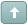Error

 Previous Topic Next Topic
 mutkan #1 Posted : Sunday, July 28, 2019 1:55:42 PM(UTC)Rank: NewbieGroups: Registered Users, Subscribers, Unverified UsersJoined: 12/14/2015(UTC)Posts: 6Thanks: 1 times I am working on Ichimoku system and need to modify it a bit. I need to figure out what the external function is doing? Can anyone help to extract the business logic running behind ExtFml("PowerPivots.SysEval"..) The forum script is provided such as ST:=(HHV(H,26)+LLV(L,26))/2; TL:=(HHV(H,9)+LLV(L,9))/2; A1:=Ref((ST+TL)/2,-25); A2:=Ref((HHV(H,52)+LLV(L,52))/2,-25); LE:= If(A1>=A2,Cross(C,A1),Cross(C,A2)); LX:= If(A1>=A2,Cross(A1,C),Cross(A2,C)); SE:= If(A1>=A2,Cross(A2,C),Cross(A1,C)); SX:= If(A1>=A2,Cross(C,A2),Cross(C,A1)); B:=ExtFml("PowerPivots.SysEval",LE,LX,SE,SX,"!@#\$#@!"); Buy:=If(TL>ST AND B>0,1,0); Sell:=If( TL < ST AND B < 0,-1,0); Buy+Sell<>-1 and Ref(Buy+Sell,-1)=-1Wanna join the discussion?! Login to your Discussions forum accountor Register a new forum account.Users browsing this topic